# Differentiation 3 Basic Rules of Differentiation The Product

• Slides: 17Differentiation 3 • Basic Rules of Differentiation • The Product and Quotient Rules • The Chain Rule • Marginal Functions in Economics • Higher-Order Derivatives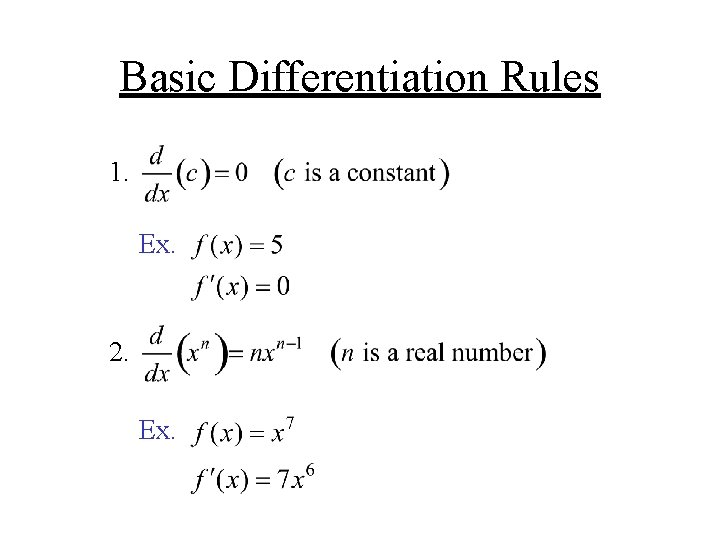Basic Differentiation Rules 1. Ex. 2. Ex.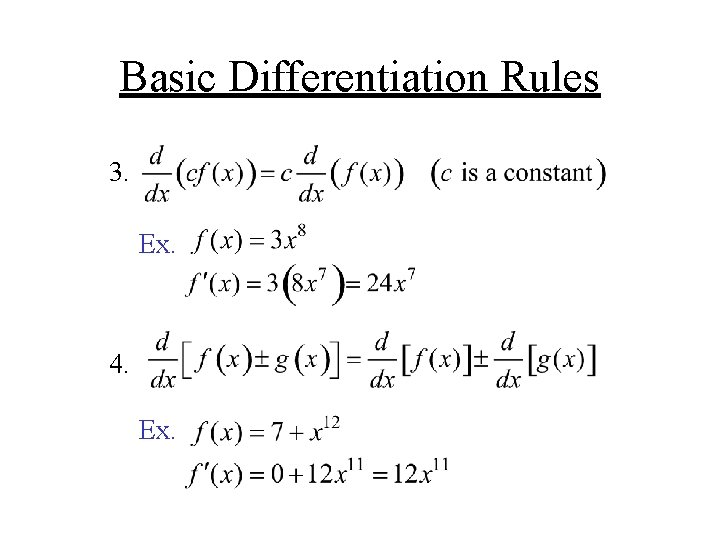Basic Differentiation Rules 3. Ex. 4. Ex.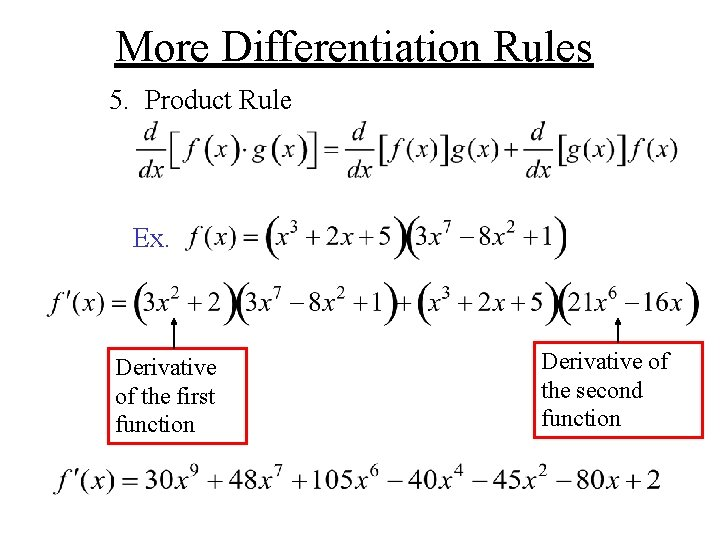More Differentiation Rules 5. Product Rule Ex. Derivative of the first function Derivative of the second functionMore Differentiation Rules 6. Quotient Rule Sometimes remembered as: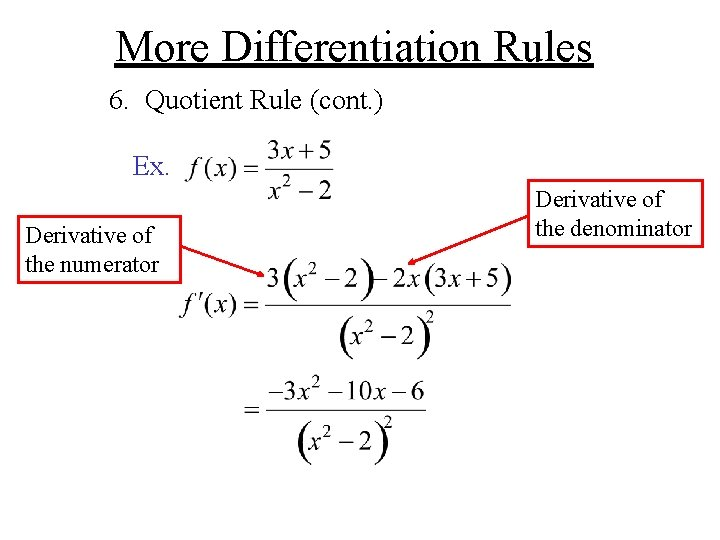More Differentiation Rules 6. Quotient Rule (cont. ) Ex. Derivative of the numerator Derivative of the denominator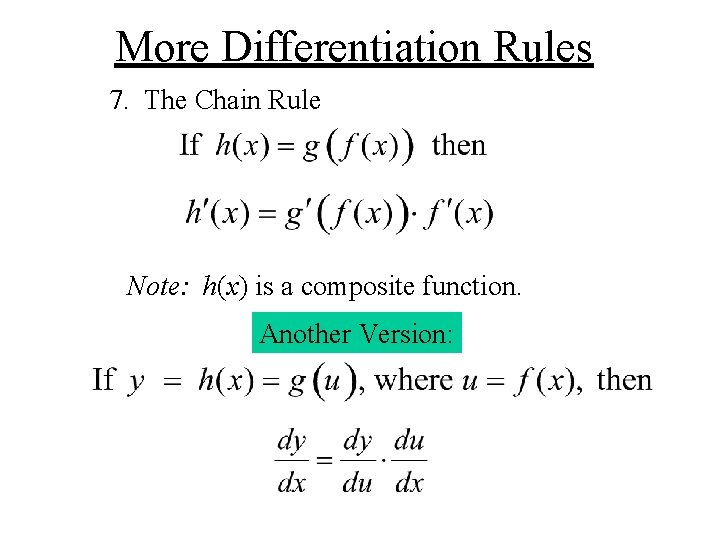More Differentiation Rules 7. The Chain Rule Note: h(x) is a composite function. Another Version:More Differentiation Rules The Chain Rule leads to The General Power Rule: Ex.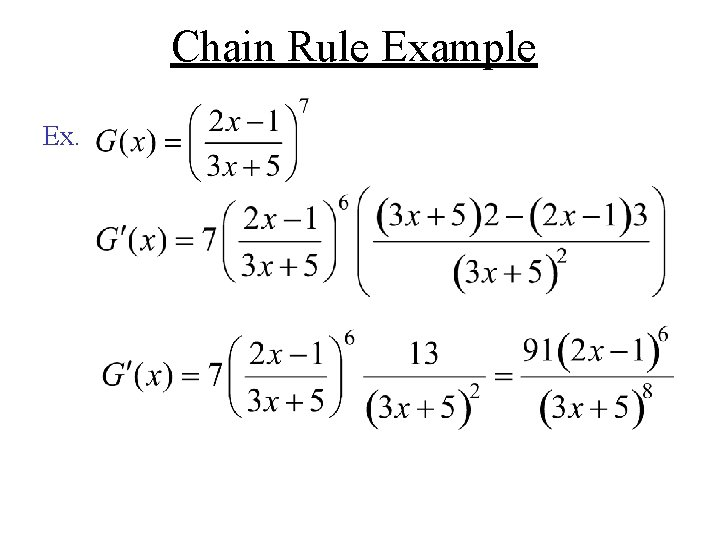Chain Rule Example Ex.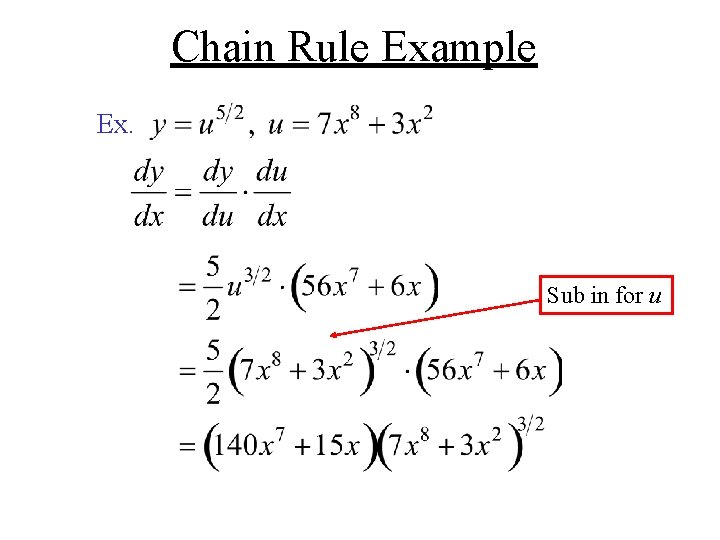Chain Rule Example Ex. Sub in for u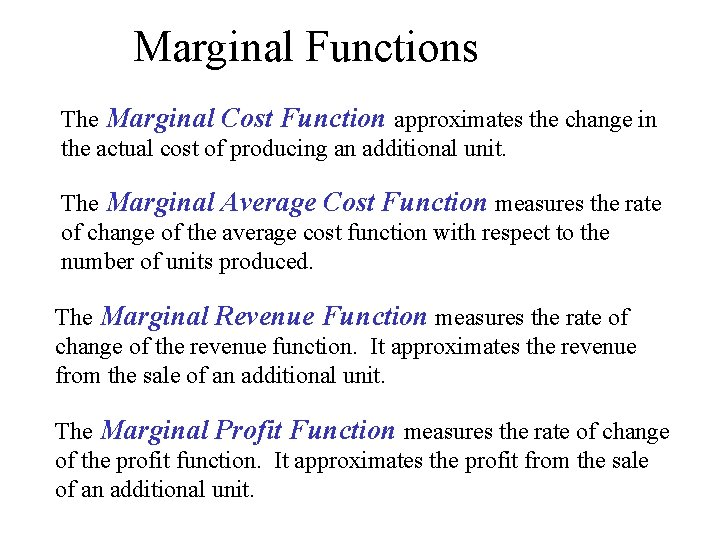Marginal Functions The Marginal Cost Function approximates the change in the actual cost of producing an additional unit. The Marginal Average Cost Function measures the rate of change of the average cost function with respect to the number of units produced. The Marginal Revenue Function measures the rate of change of the revenue function. It approximates the revenue from the sale of an additional unit. The Marginal Profit Function measures the rate of change of the profit function. It approximates the profit from the sale of an additional unit.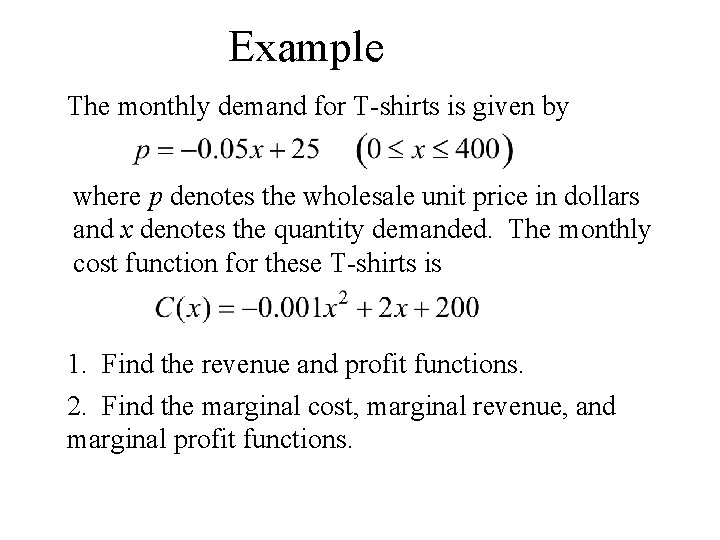Example The monthly demand for T-shirts is given by where p denotes the wholesale unit price in dollars and x denotes the quantity demanded. The monthly cost function for these T-shirts is 1. Find the revenue and profit functions. 2. Find the marginal cost, marginal revenue, and marginal profit functions.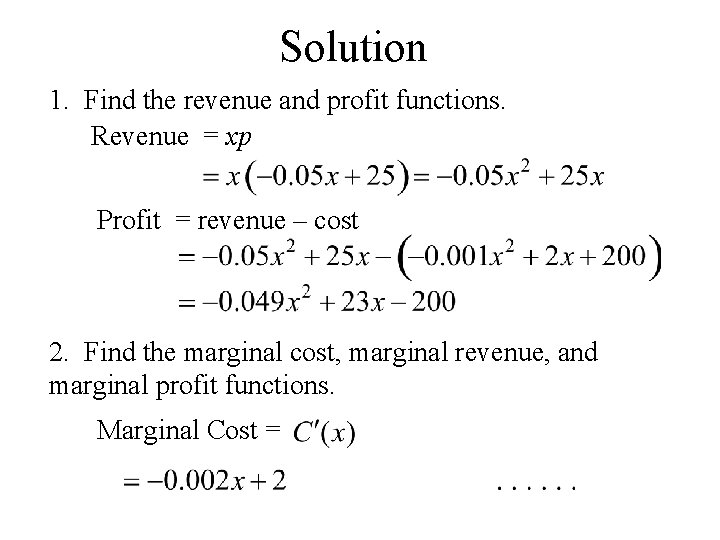Solution 1. Find the revenue and profit functions. Revenue = xp Profit = revenue – cost 2. Find the marginal cost, marginal revenue, and marginal profit functions. Marginal Cost =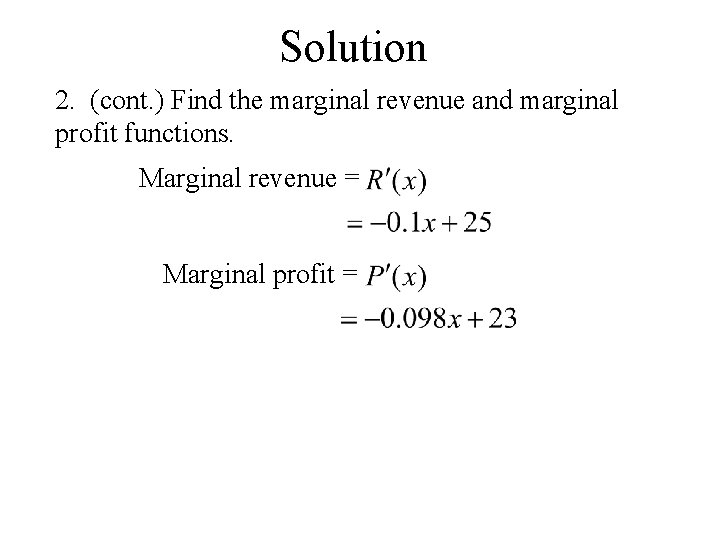Solution 2. (cont. ) Find the marginal revenue and marginal profit functions. Marginal revenue = Marginal profit =Higher Derivatives The second derivative of a function f is the derivative of f at a point x in the domain of the first derivative. Derivative Second Third Fourth nth NotationsExample of Higher Derivatives Given findExample of Higher Derivatives Given find